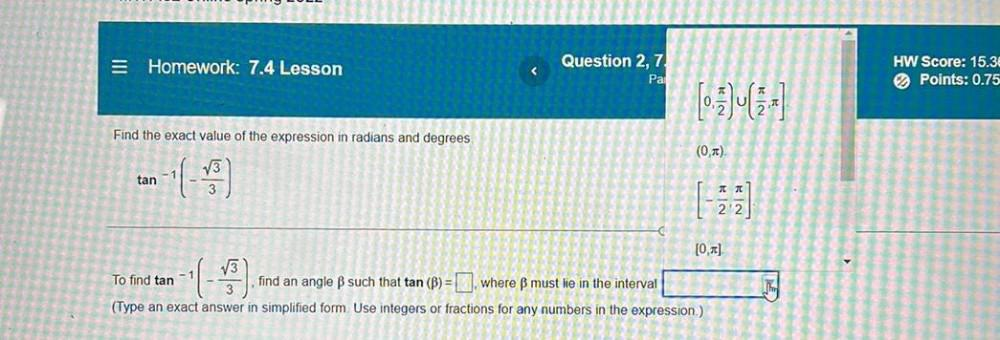Question:

# = Homework: 7.4 Lesson Question 2,7 Pa HW Score: 15.3 Points: 0.75 Find the exact value of the expression in radians and degrees= Homework: 7.4 Lesson Question 2,7 Pa HW Score: 15.3 Points: 0.75 Find the exact value of the expression in radians and degrees (0) tan JT 22 [O] To find tan - 1 find an angle B such that tan (B) = where ß must lie in the interval (Type an exact answer in simplified form Use integers or fractions for any numbers in the expression) ww